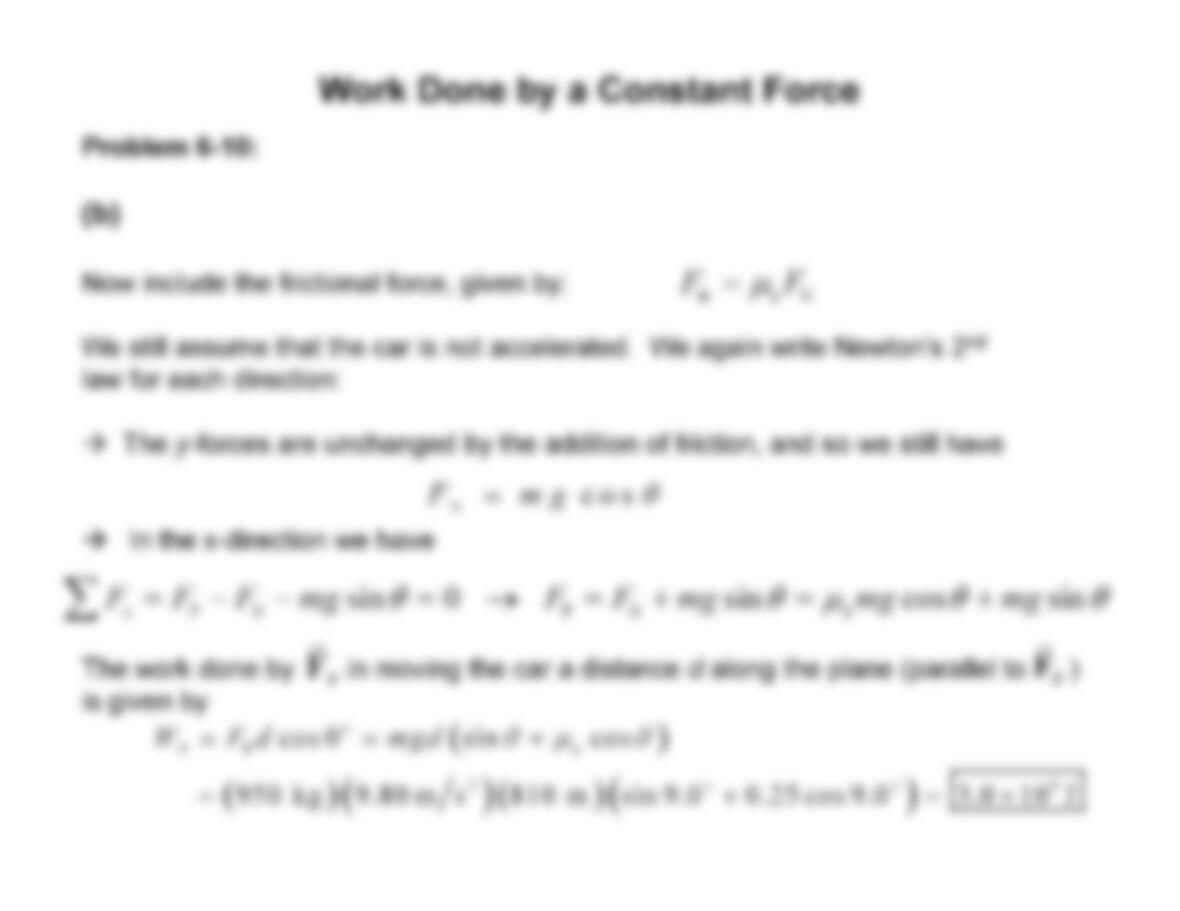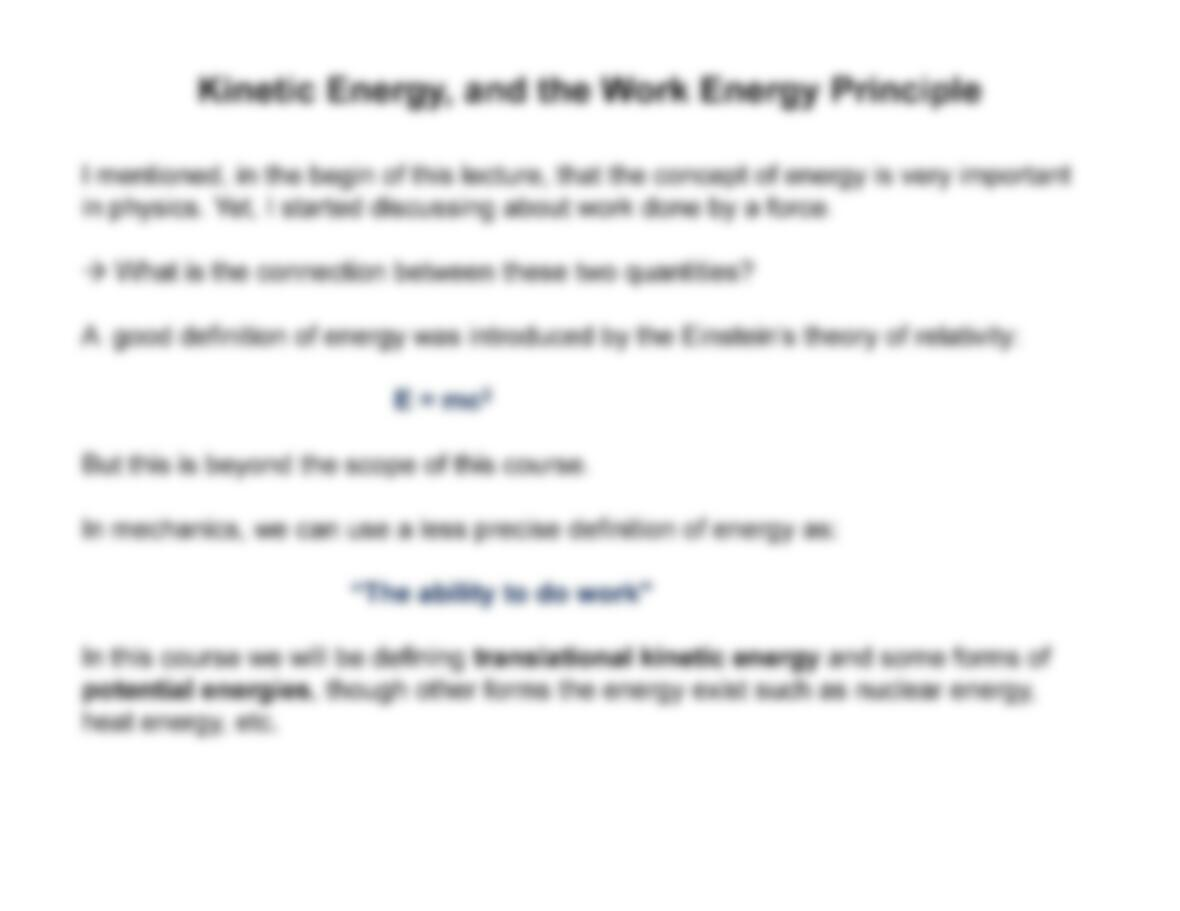Type
Essay
Pages
19 pages
Word Count
2051 words
School
Course Code
physics

# Physics

November 18, 2020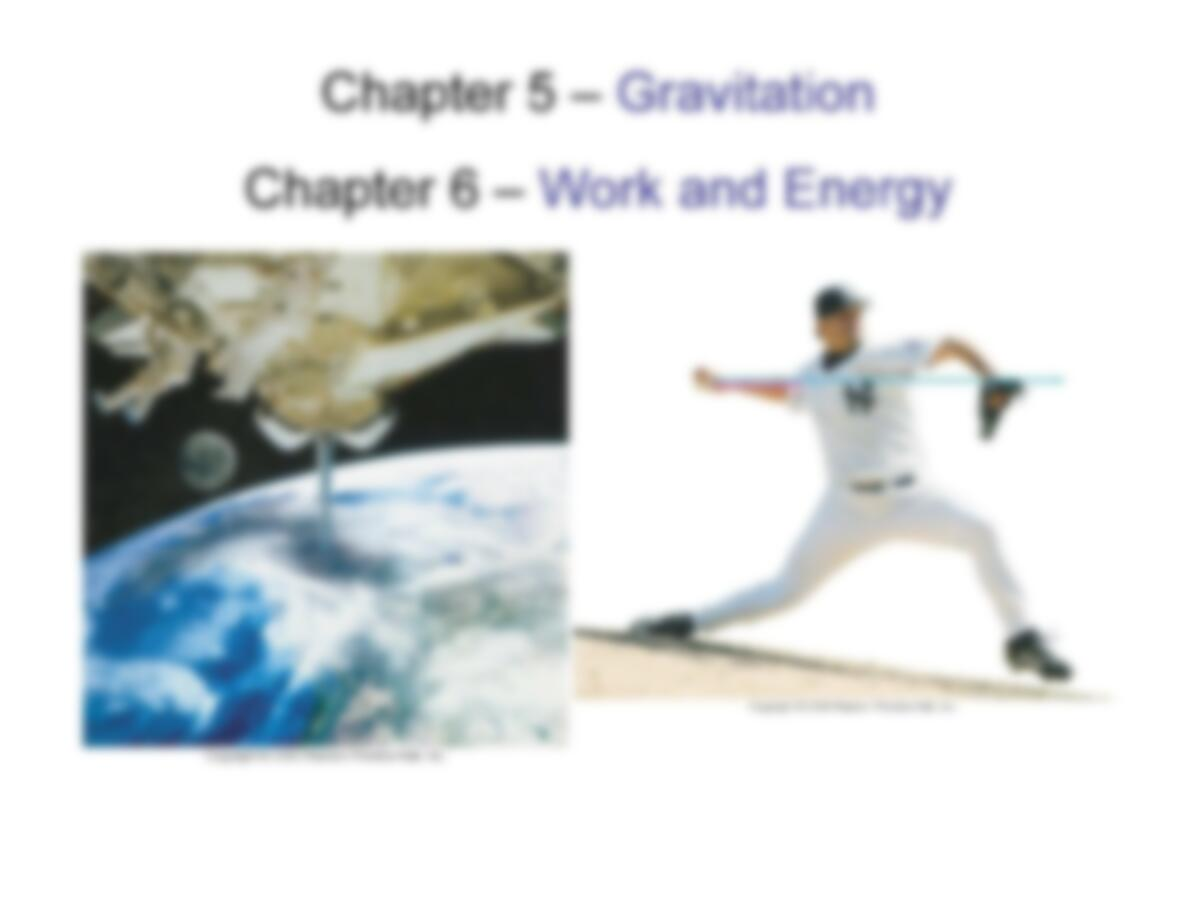Chapter 5 – Gravitation
Chapter 6 – Work and EnergyChapter 5
• (5.6) Newton’s Law of Universal Gravitation
• (5.7) Gravity Near the Earth’s Surface
Chapter 6 (today)
• Work Done by a Constant Force
• Kinetic Energy, and the Work-Energy PrincipleOld assignments can be picked up
in my office
(LB-212)Recalling Recalling
LastLast
LectureLecture
Recalling Recalling
LastLast
LectureLectureNewton’s Law of Universal GravitationNewton’s Law of Universal Gravitation
Newton’s law of universal gravitation:
Every particle in the universe attracts every other particle with a force that is
proportional to the products of their masses and inversely proportional to the
square of the distance between them. This forces acts along the line joining the
square of the distance between them. This forces acts along the line joining the
two particles.”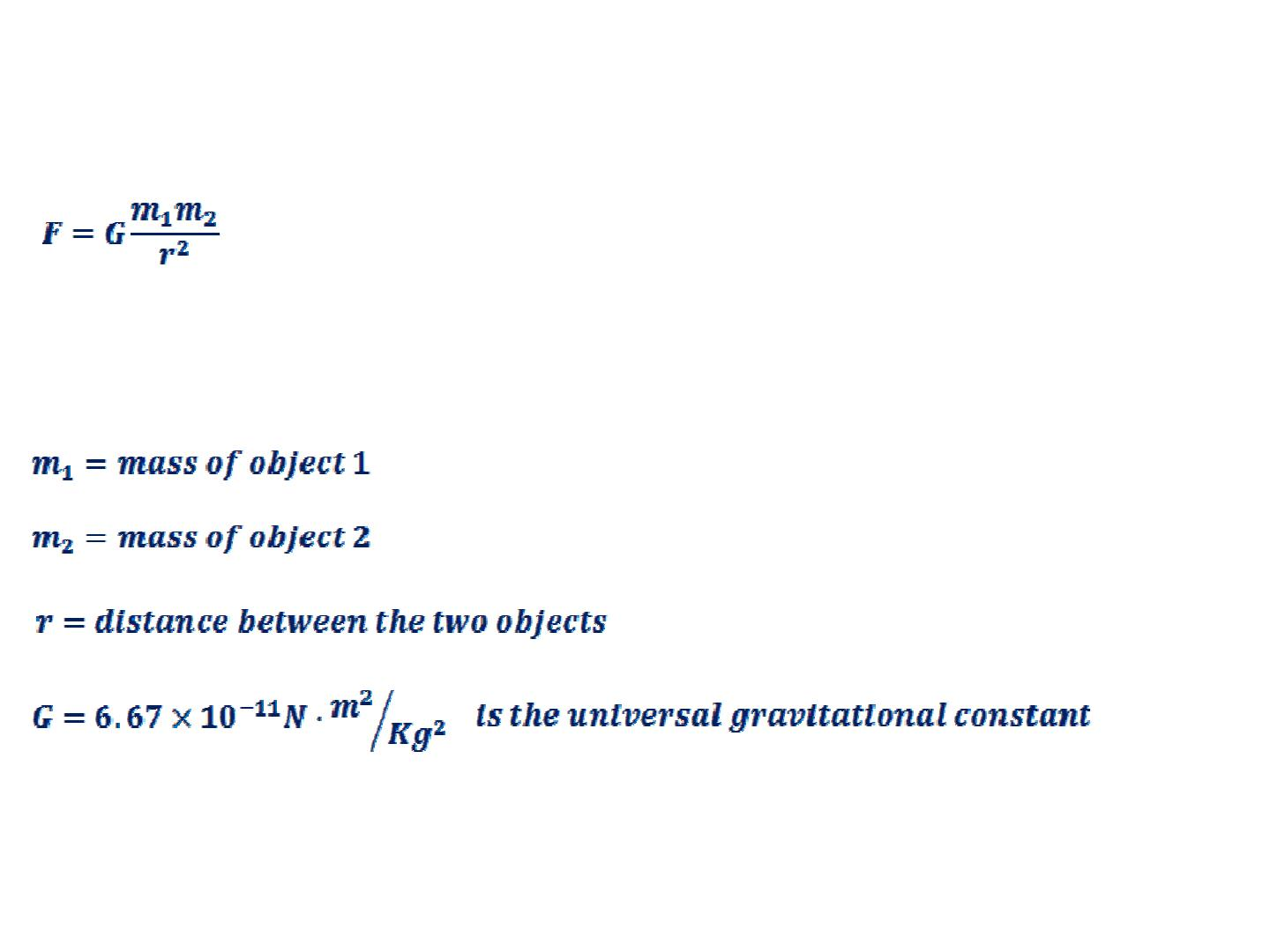Newton’s Law of Universal GravitationNewton’s Law of Universal Gravitation
The magnitude of this force is given by:
Where:
(4.7)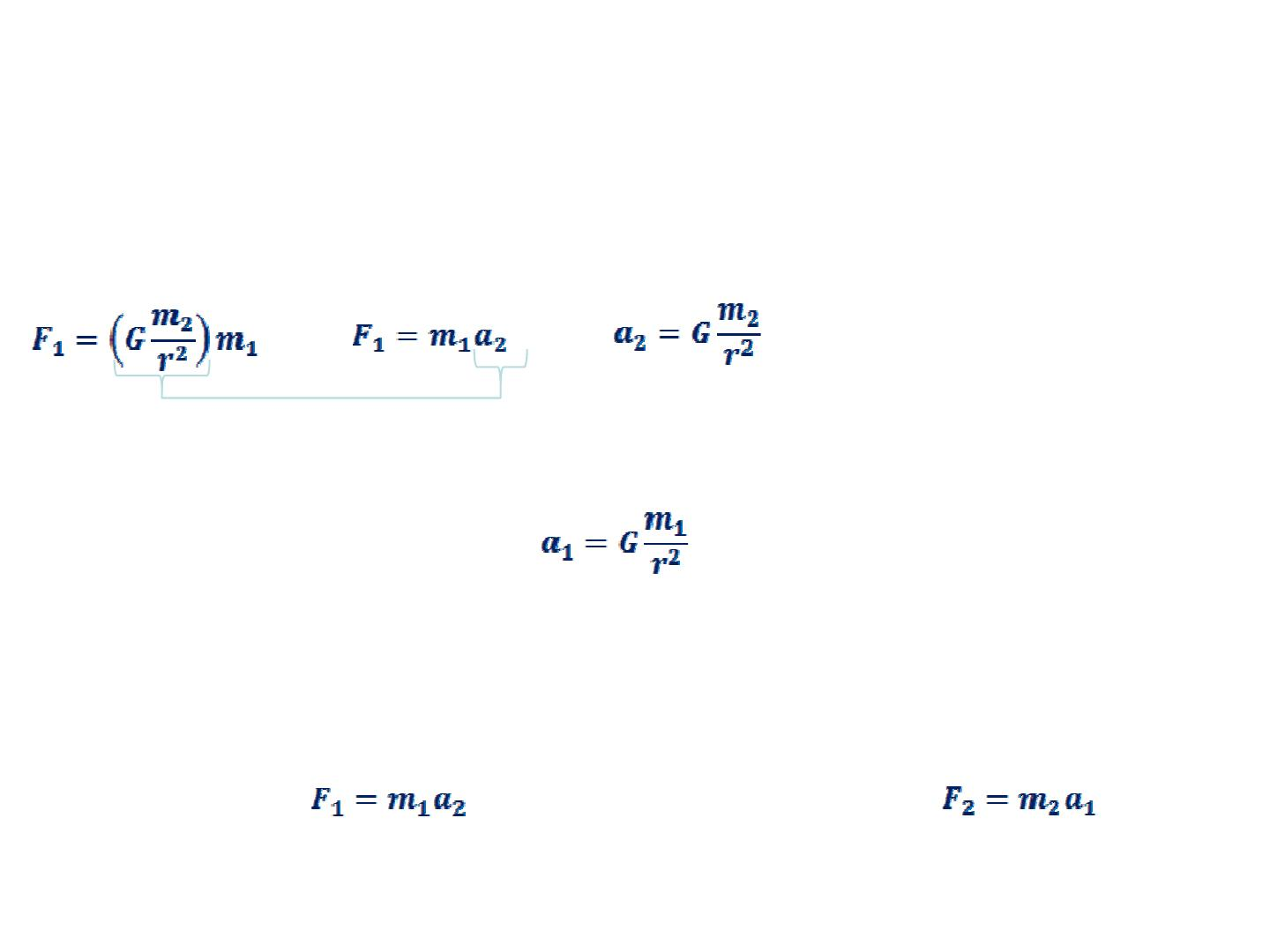Newton’s Law of Universal GravitationNewton’s Law of Universal Gravitation
If m2is the object exerting a force on m1, then the gravitational acceleration felt
by object 1 can be identified as:
And vice-versa: object 2 will feel an acceleration
(4.8)
due to the gravitational force applied by object 1.
You can then write for the force acting on m1and for the
force acting on m2.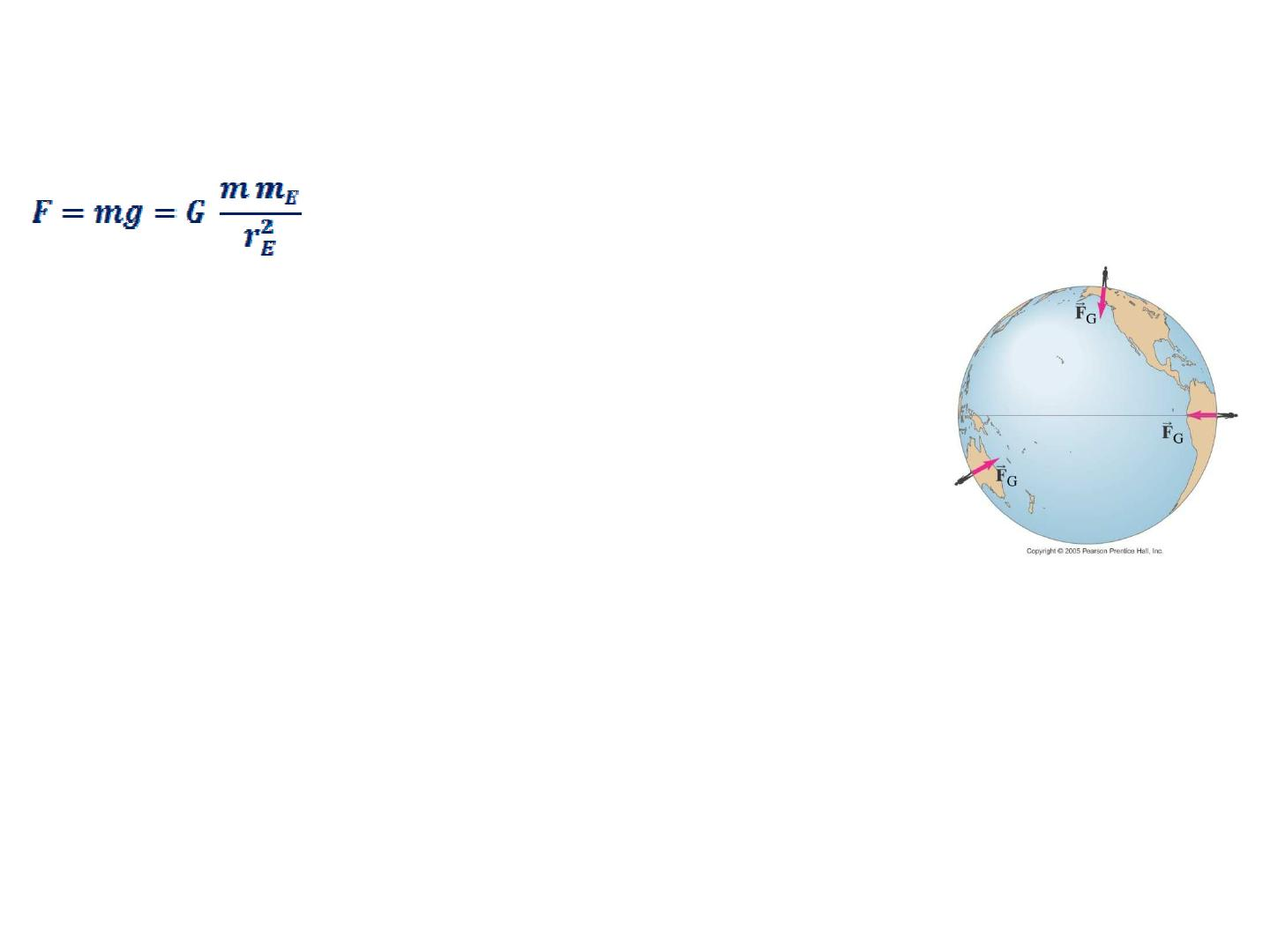Gravity Near the Earth’s SurfaceGravity Near the Earth’s Surface
An object of mass mON the surface of the Earth will feel a force given by:
Where,
mE= mass of the Earth ;
r
E
= 6.38 x 10
6
.
(4.9)
r
E
= 6.38 x 10
6
.
ghas been measured and it is known to be 9.80 m/s2.
In fact, the value of g can be considered constant at any position near the Earth’s
surface
Accurate measurements yield: mE= 5.974 x 1024 KgNewton’s Law of Universal GravitationNewton’s Law of Universal Gravitation
Problem 5-31 (textbook): A hypothetical planet has a radius 1.5 times that of Earth,
but has the same mass. What is the acceleration due to gravity near its surface?
Solution:
The acceleration due to gravity at any location on or above the surface of a planet is
given by
2
planet Planet
g G M r
=
where ris the distance from the center of the planet to the location in question.
( )
2
2
Planet Earth Earth
planet Earth
2
2 2 2 2 2
Earth
Earth
1 1 9.8 m s
4.4 m s
1.5 1.5 1.5
1.5
M M M
g G G G g
r R
R
= = = = = =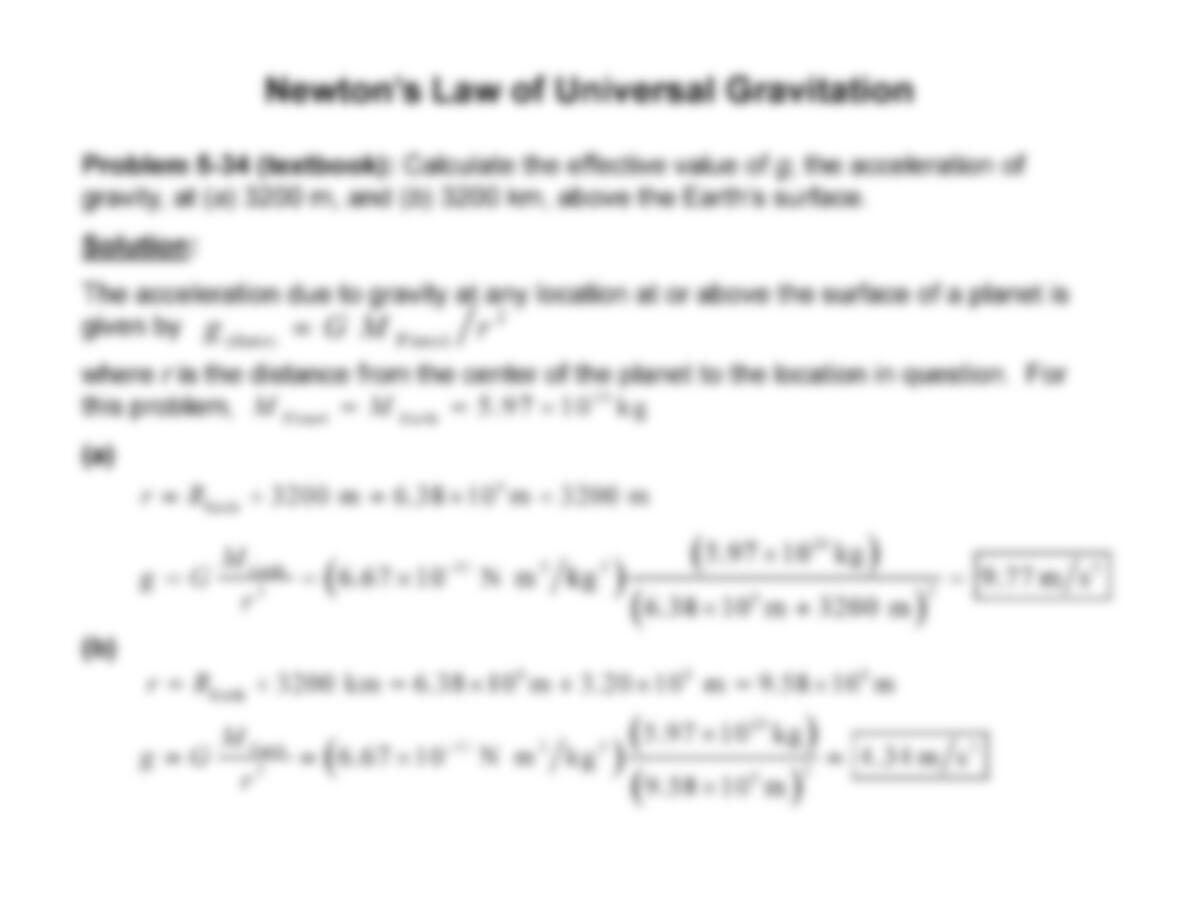Newton’s Law of Universal GravitationNewton’s Law of Universal Gravitation
Problem 5-34 (textbook): Calculate the effective value of g, the acceleration of
gravity, at (a) 3200 m, and (b) 3200 km, above the Earth’s surface.
Solution: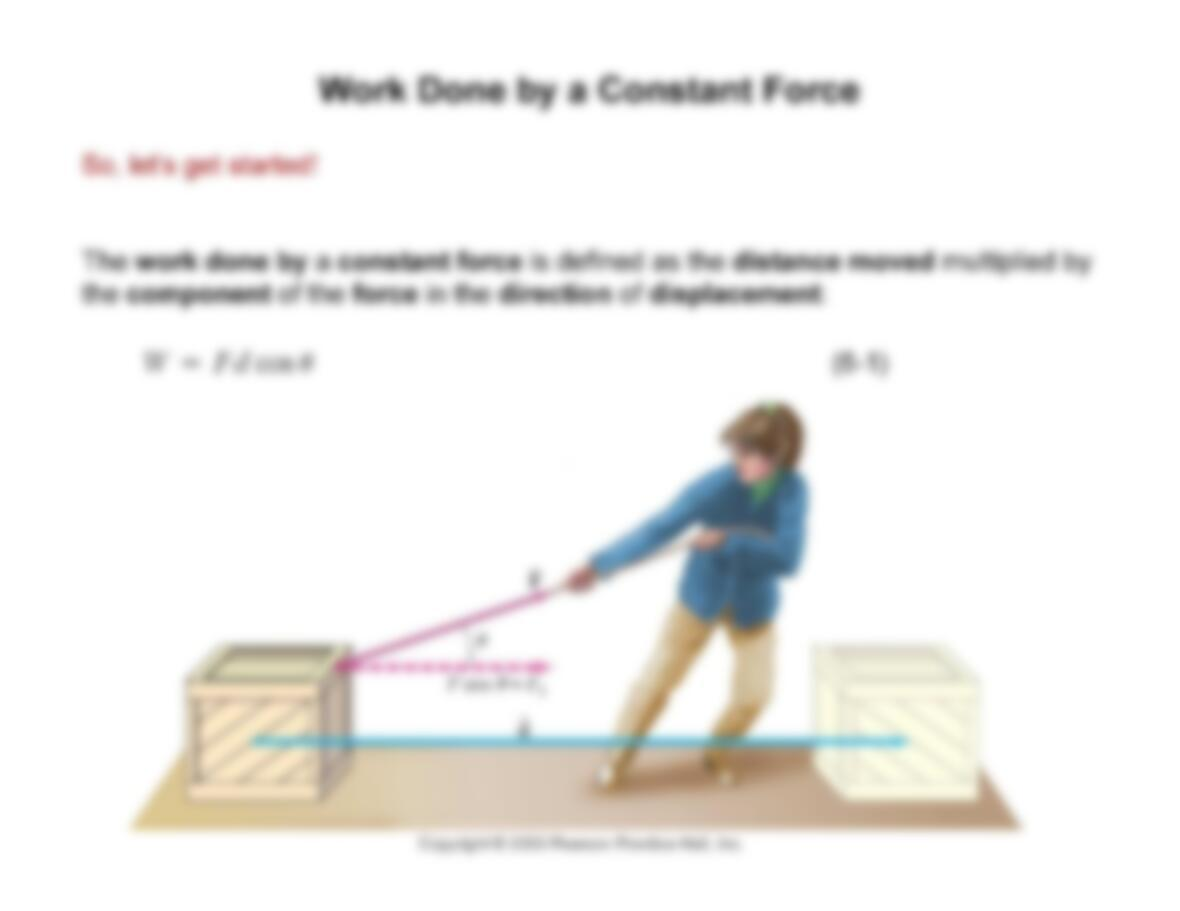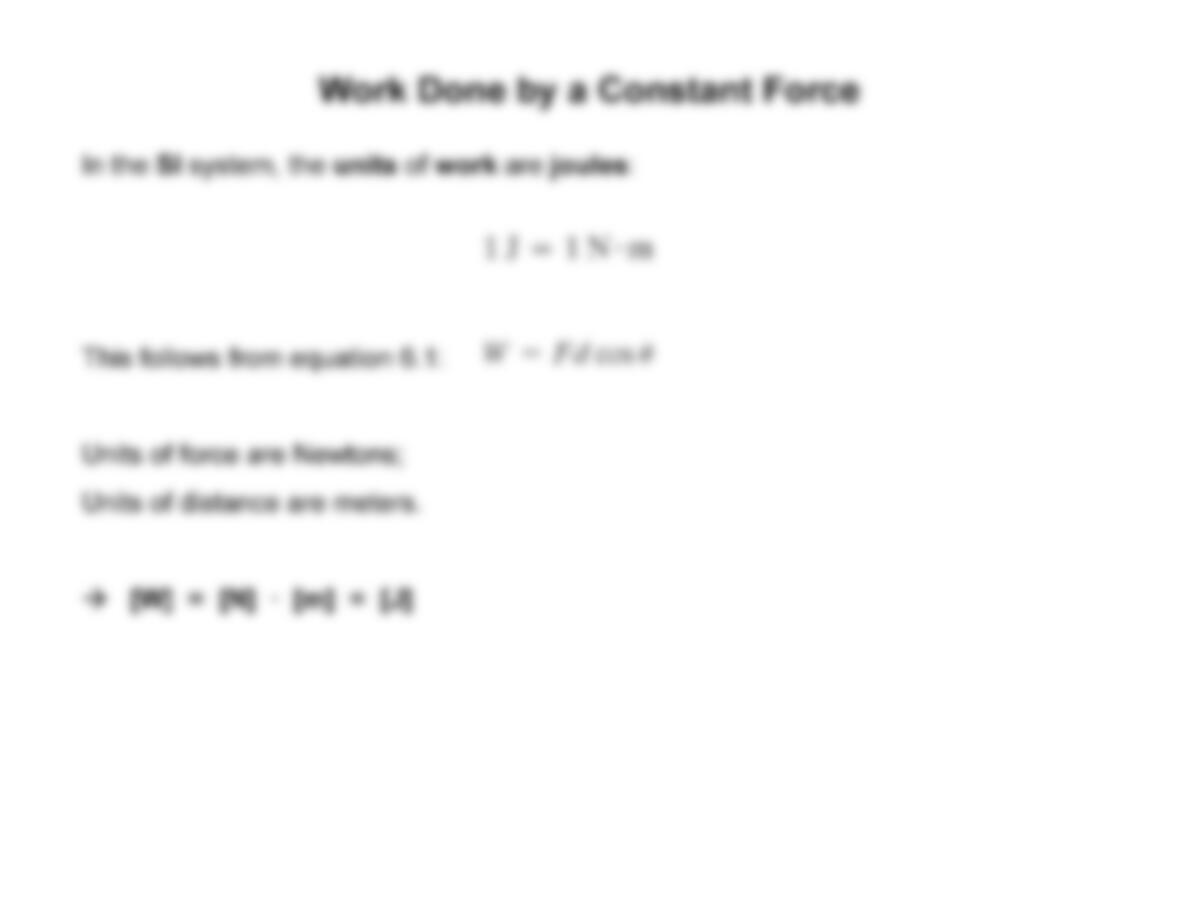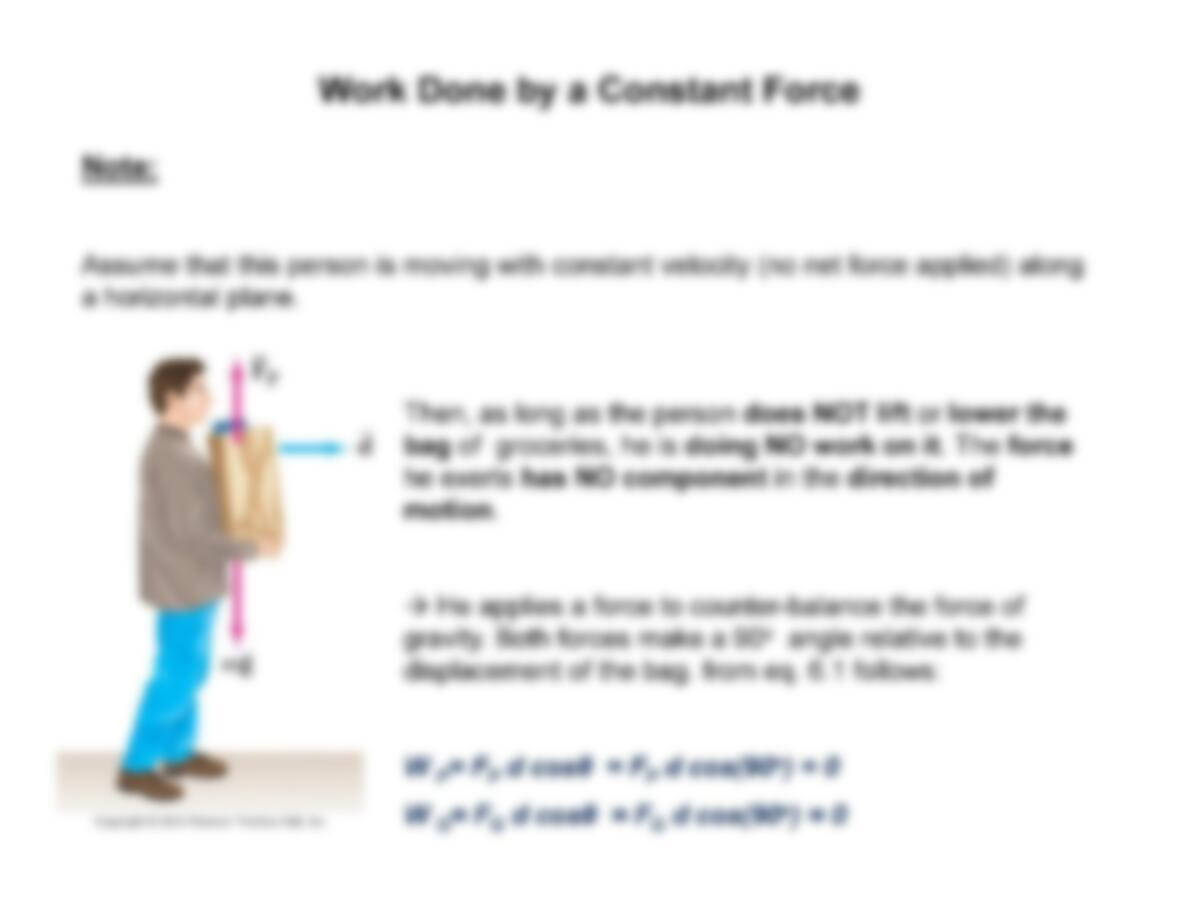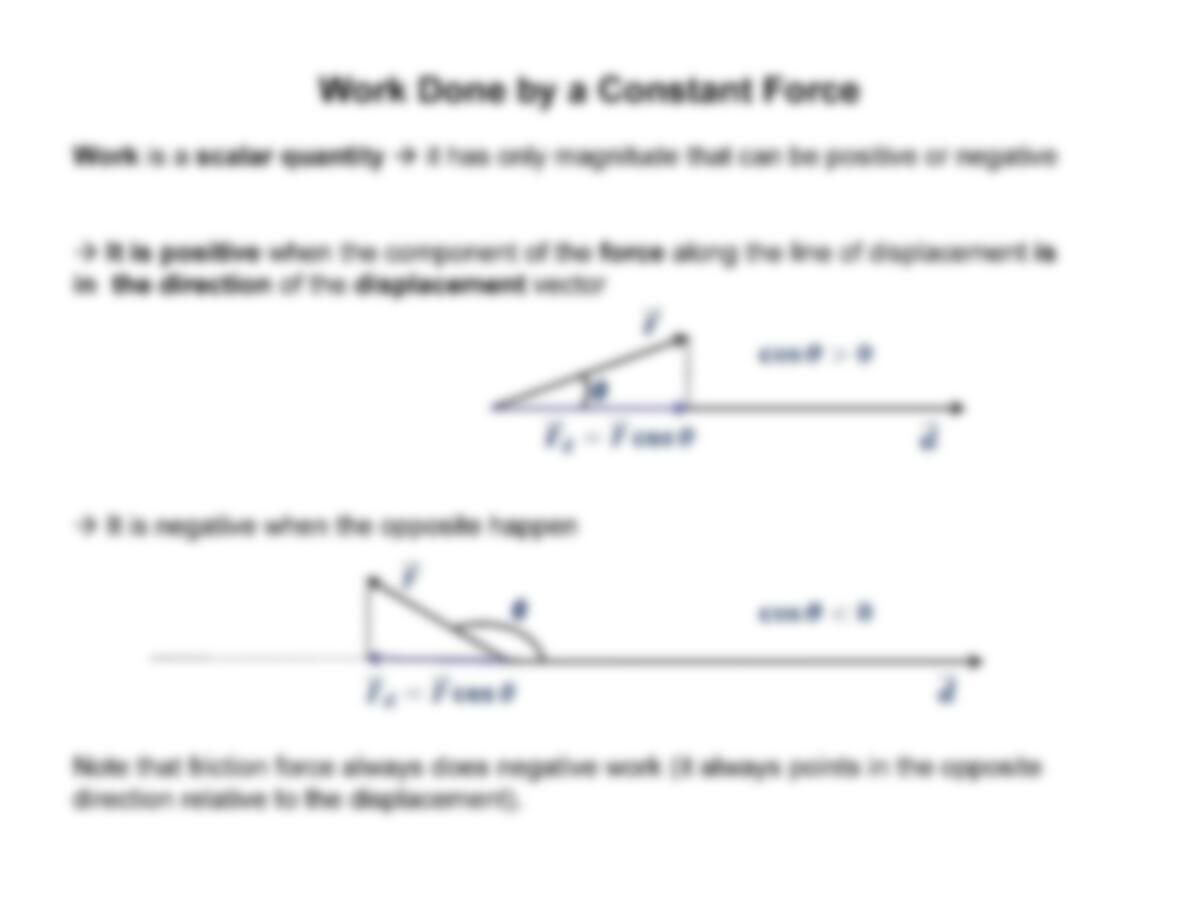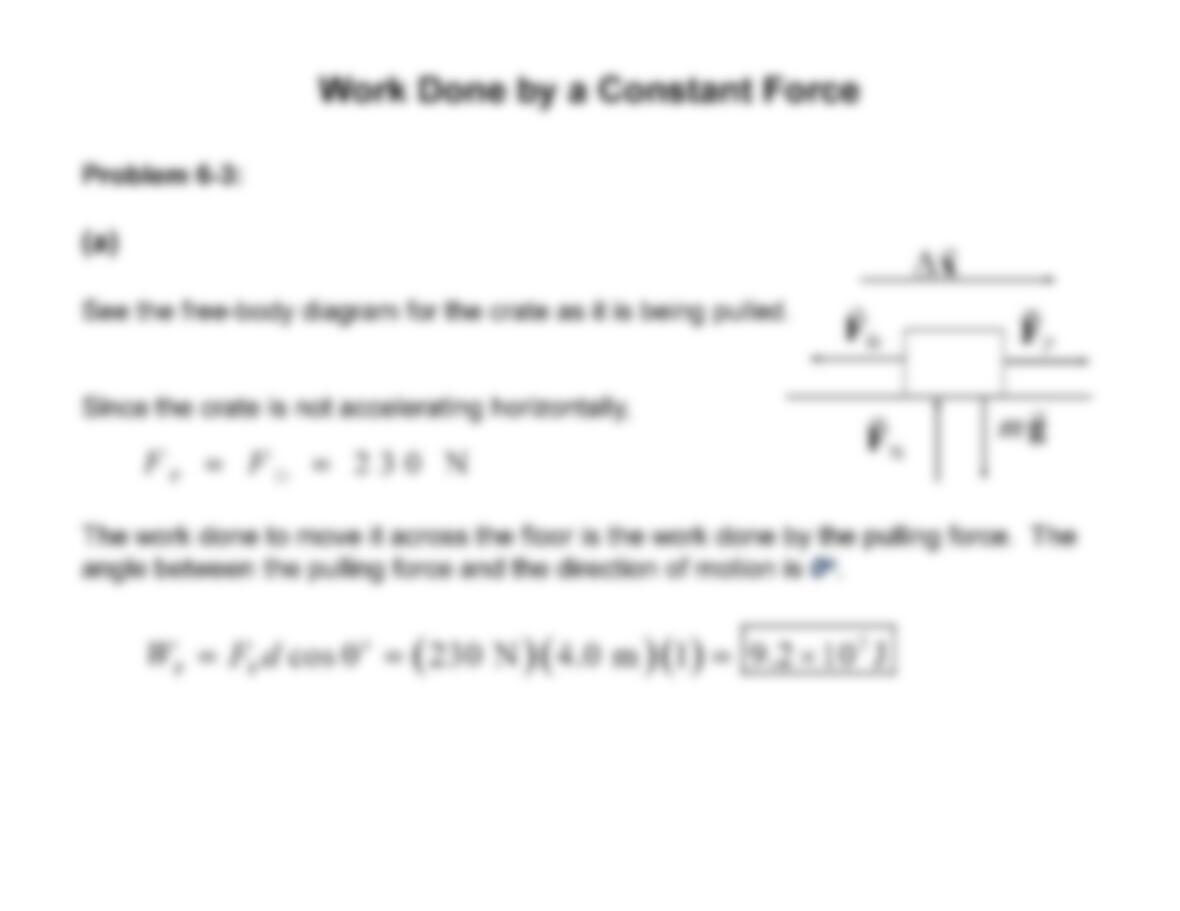P P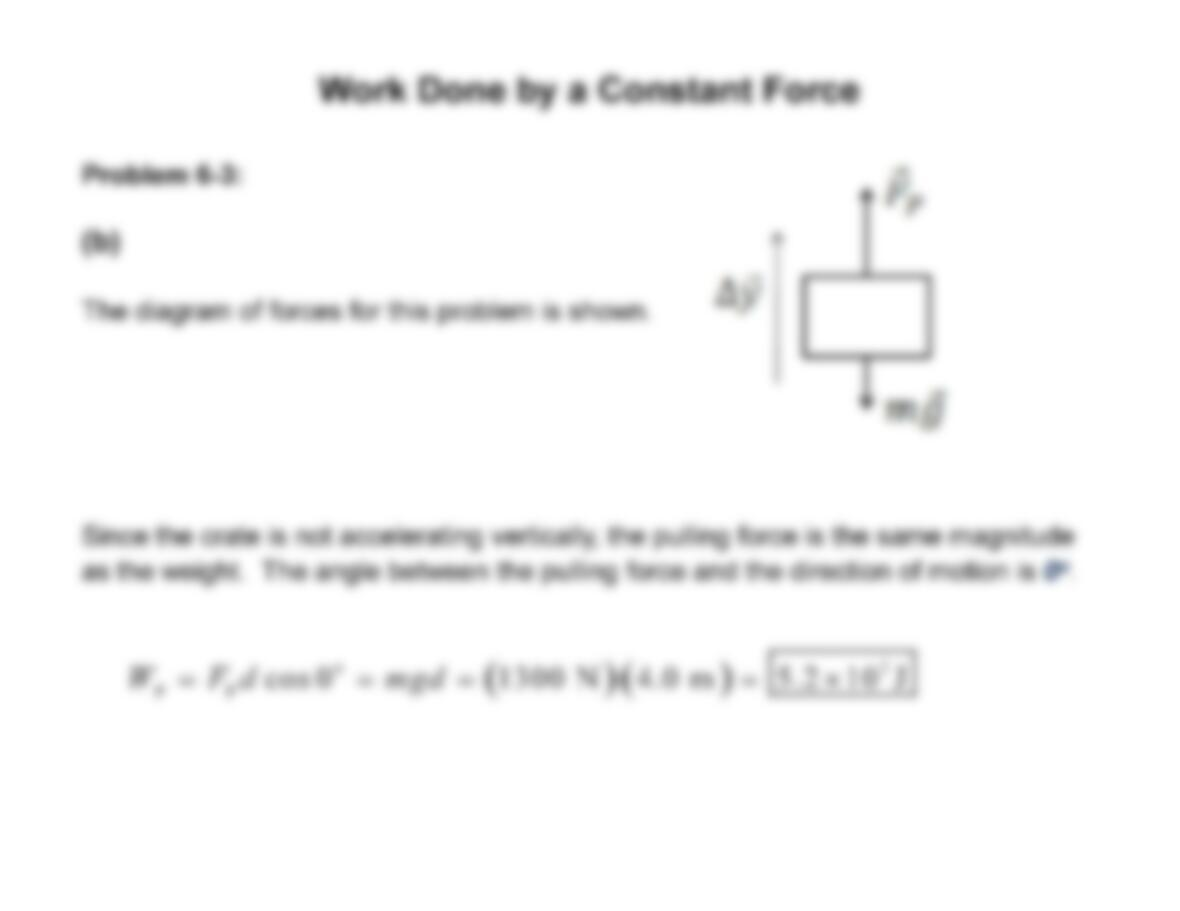P P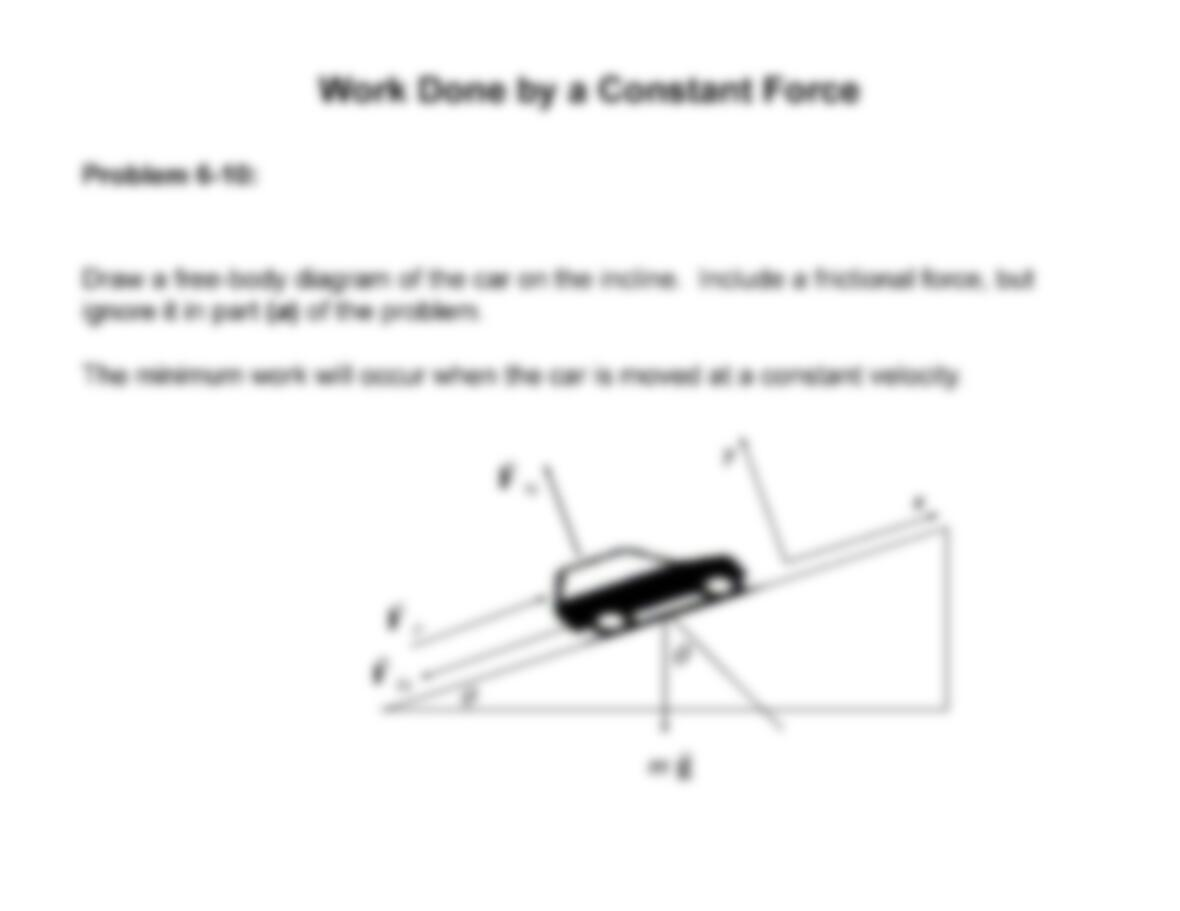y
θ
θ
y
x
m
g
r
N
F
r
f r
F
r
P
F
r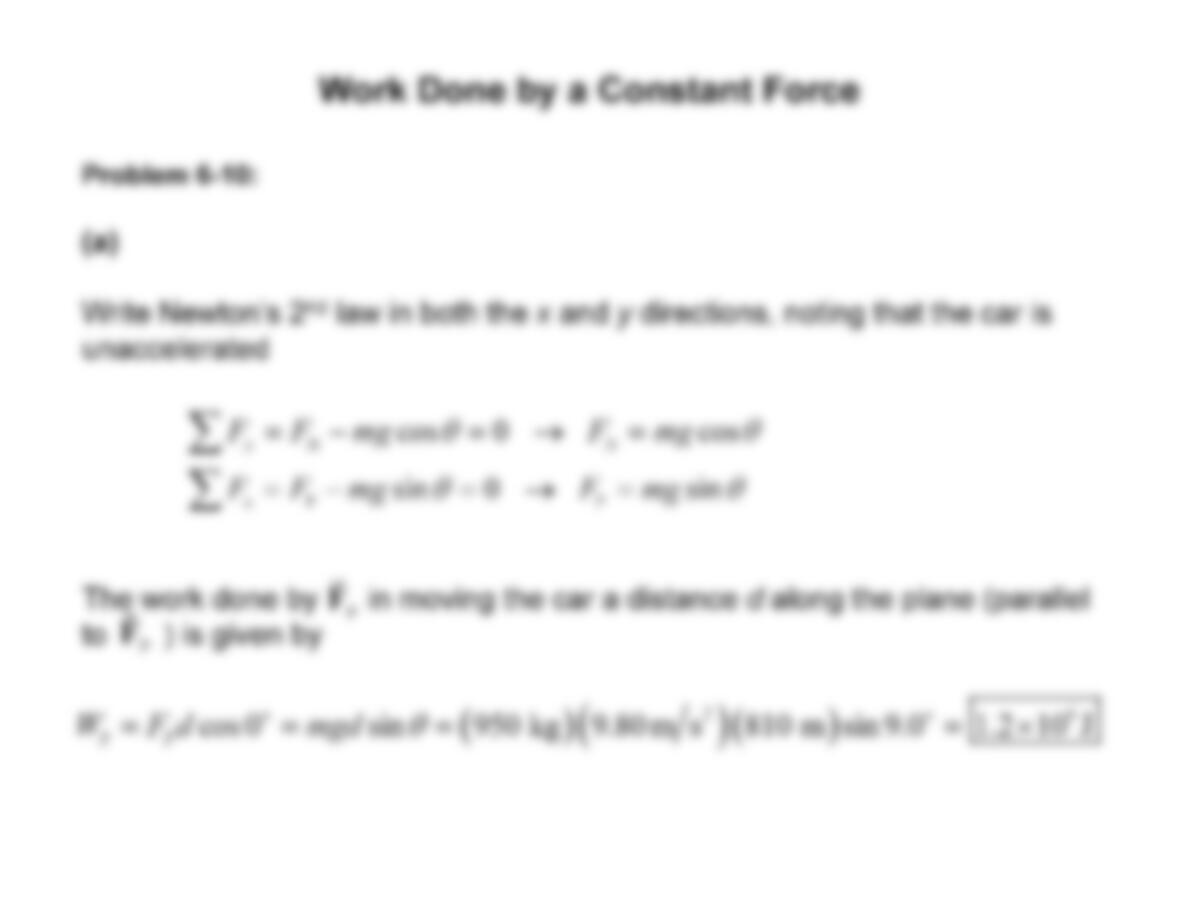P P